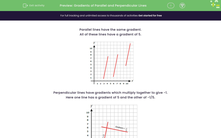# Gradients of Parallel and Perpendicular Lines

In this worksheet, students answer questions using the relationship between the gradients of parallel and perpendicular lines.Key stage:  KS 3

Curriculum topic:   Algebra

Curriculum subtopic:   Use Linear Equations (Two Variables) and Gradients

Difficulty level:#### Worksheet Overview

Parallel lines have the same gradient.

All of these lines have a gradient of 5.Perpendicular lines have gradients which multiply together to give -1.

Here one line has a gradient of 5 and the other of -1/5.To find the gradient of a line which is perpendicular to one of gradient a/b, we invert the fraction and multiply by -1.

This means you don't need diagrams to check if lines are perpendicular.

Example

State the gradient of a line which is perpendicular to one of gradient ¾.

Invert the fraction and multiply by -1.

 3 → 4 → -4 4 3 3